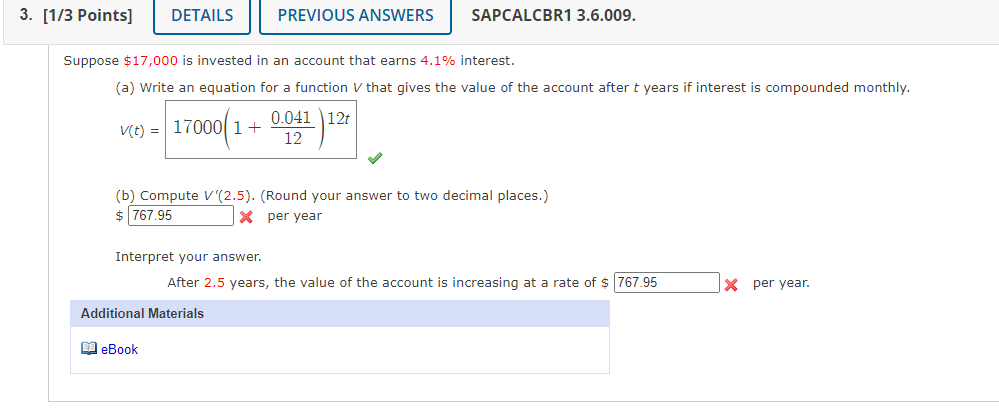Home / Expert Answers / Calculus / suppose-17-000-is-invested-in-an-account-that-earns-4-1-interest-a-write-an-equation-for-a-fu-pa302

# (Solved): Suppose \$17,000 is invested in an account that earns 4.1% interest. (a) Write an equation for a fu ...Suppose is invested in an account that earns interest. (a) Write an equation for a function that gives the value of the account after years if interest is compounded monthly. (b) Compute . (Round your answer to two decimal places.) per year Interpret your answer. After 2.5 years, the value of the account is increasing at a rate of per year.

We have an Answer from Expert